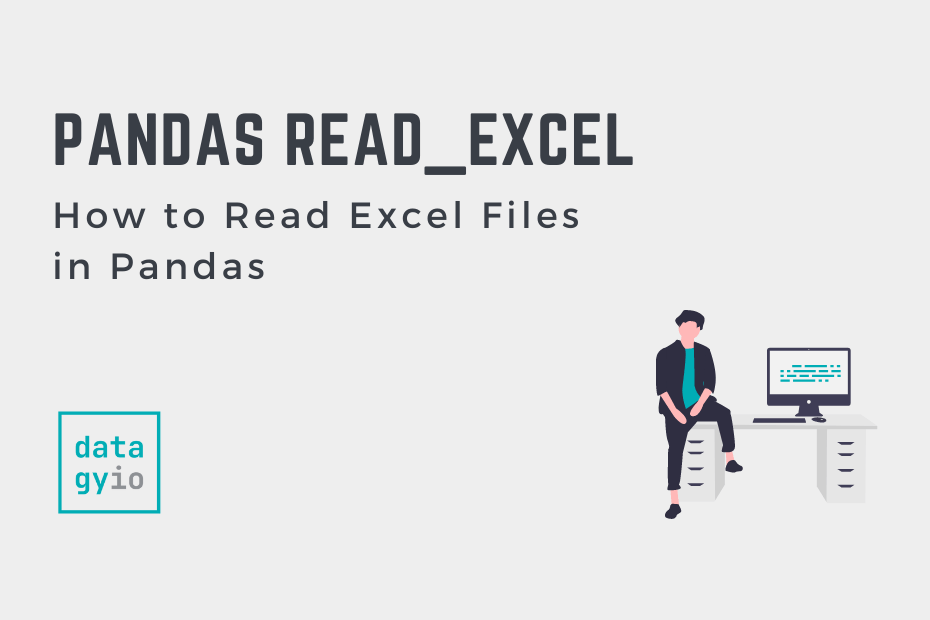# Pandas Tutorials## How to Use Pandas to Read Excel Files in Python

In this tutorial, you’ll learn how to use Python and Pandas to read Excel files using the Pandas read_excel function. Excel files are everywhere – and while they may not be the ideal data type for many data scientists, knowing… Read More »How to Use Pandas to Read Excel Files in Python## Convert a List of Dictionaries to a Pandas DataFrame

In this tutorial, you’ll learn how to convert a list of Python dictionaries into a Pandas DataFrame. Pandas provides a number of different ways in which to convert dictionaries into a DataFrame. You’ll learn how to use the Pandas from_dict… Read More »Convert a List of Dictionaries to a Pandas DataFrame## How to Add / Insert a Row into a Pandas DataFrame

In this tutorial, you’ll learn how to add (or insert) a row into a Pandas DataFrame. You’ll learn how to add a single row, multiple rows, and at specific positions. You’ll also learn how to add a row using a… Read More »How to Add / Insert a Row into a Pandas DataFrame## Pandas Scatter Plot: How to Make a Scatter Plot in Pandas

In this tutorial, you’ll learn how to use Pandas to make a scatter plot. Under the hood, Pandas uses Matplotlib, which can make customizing your plot a familiar experience. Pandas allows you to customize your scatter plot by changing colors,… Read More »Pandas Scatter Plot: How to Make a Scatter Plot in Pandas## Pandas to_datetime: Convert a Pandas String Column to Date Time

In this tutorial, you’ll learn how to use the Pandas to_datetime function to convert a Pandas column to date time. Pandas provides a huge number of methods and functions that make working with dates incredibly versatile. However, data aren’t always… Read More »Pandas to_datetime: Convert a Pandas String Column to Date Time## Introduction to Pandas for Data Science

In this tutorial, you’ll learn how to dive into the wonderful world of Pandas. Pandas is a Python package that provides fast and flexible data structures used for data manipulation and analysis. By the end of this tutorial, you’ll have… Read More »Introduction to Pandas for Data Science## Indexing, Selecting, and Assigning Data in Pandas

In this tutorial, you’ll learn how to index, select and assign data in a Pandas DataFrame. Understanding how to index and select data is an important first step in almost any exploratory work you’ll take on in data science. Similarly,… Read More »Indexing, Selecting, and Assigning Data in Pandas## Summarizing and Analyzing a Pandas DataFrame

In this tutorial, you’ll learn how to quickly summarize and analyze a Pandas DataFrame. By the end of this tutorial, you’ll have learned to take on some exploratory analysis of your dataset using pandas. You’ll learn how to calculate general… Read More »Summarizing and Analyzing a Pandas DataFrame## Transforming Pandas Columns with map and apply

In this tutorial, you’ll learn how to transform your Pandas DataFrame columns using vectorized functions and custom functions using the map and apply methods. By the end of this tutorial, you’ll have a strong understanding of how Pandas applies vectorized… Read More »Transforming Pandas Columns with map and apply## Binning Data in Pandas with cut and qcut

In this tutorial, you’ll learn how to bin data in Python with the Pandas cut and qcut functions. You’ll learn why binning is a useful skill in Pandas and how you can use it to better group and distill information.… Read More »Binning Data in Pandas with cut and qcut## DateTime in Pandas and Python

In this tutorial, you’ll learn how to work with dates, times, and DateTime in Pandas and Python. Working with DateTime in Python and Pandas can be a complicated thing. This guide aims to make the complicated, simple, by focusing on… Read More »DateTime in Pandas and Python## Data Cleaning and Preparation in Pandas and Python

In this tutorial, you’ll learn how to clean and prepare data in a Pandas DataFrame. You’ll learn how to work with missing data, how to work with duplicate data, and dealing with messy string data. Being able to effectively clean… Read More »Data Cleaning and Preparation in Pandas and Python